# Abelian variety

(diff) ← Older revision | Latest revision (diff) | Newer revision → (diff)
The theory of Abelian varieties over the field of complex numbersis, in essence, equivalent to the theory of Abelian functions founded by C.G.J. Jacobi, N.H. Abel and B. Riemann. If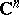denotes-dimension vector space,is a lattice (cf. Discrete subgroup) of rank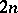, then the quotient group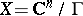is a complex torus. Meromorphic functions onare the same thing as meromorphic functions onthat are invariant with respect to the period lattice. If the fieldof meromorphic functions onhas transcendence degree, thencan be given the structure of an algebraic group. This structure is unique by virtue of the compactness of, and it is such that the field of rational functions of this structure coincides with. The algebraic groups formed in this way are Abelian varieties, and each Abelian variety over the fieldarises in this way. The matrix which defines a basis ofcan be reduced to the form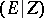, where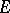is the identity matrix and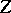is a matrix of order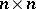. The complex torusis an Abelian variety if and only ifis symmetric and has positive-definite imaginary part. It should be pointed out that, as real Lie groups, all varietiesare isomorphic, but this is not true for their analytic or algebraic structures, which vary strongly when deforming the lattice. Inspection of the period matrixshows that its variation has an analytic character, which results in the construction of the moduli variety of all Abelian varieties of given dimension. The dimension of the moduli variety is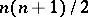(cf. Moduli problem).
The theory of Abelian varieties over an arbitrary fieldis due to A. Weil , . It has numerous applications both in algebraic geometry itself and in other fields of mathematics, particularly in number theory and in the theory of automorphic functions. To each complete algebraic variety, Abelian varieties (cf. Albanese variety; Picard variety; Intermediate Jacobian) can be functorially assigned. These constructions are powerful tools in studying the geometric structures of algebraic varieties. E.g., they were used to obtain one of the solutions of the Lüroth problem. Another application is the proof of the Riemann hypothesis for algebraic curves over a finite field — the problem for which the abstract theory of Abelian varieties was originally developed. It was also one of the sources of-adic cohomology. The simplest example of such a cohomology is the Tate module of an Abelian variety. It is the projective limit, as, of the groups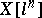of points of order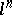. The determination of the structure of such groups was one of the principal achievements of the theory of Weil. In fact, ifis coprime with the characteristicof the fieldand ifis algebraically closed, then the group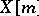is isomorphic to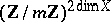. If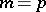, the situation is more complicated, which resulted in the appearance of concepts such as finite group schemes, formal groups and-divisible groups (cf. Finite group scheme; Formal group;-divisible group). The study of the action of endomorphisms of Abelian varieties, in particular of the Frobenius endomorphism on its Tate module, makes it possible to give a proof of the Riemann hypothesis (for algebraic curves over finite fields, cf. Riemann hypotheses) and is also the principal instrument in the theory of complex multiplication of Abelian varieties. Another circle of problems connected with the Tate module consists of a study of the action of the Galois group of the closure of the ground field on this module. There resulted the Tate conjectures and the theory of Tate–Honda, which describes Abelian varieties over finite fields in terms of the Tate module .
The study of Abelian varieties over local fields, including-adic fields, is proceeding at a fast rate. An analogue of the above-mentioned representation of Abelian varieties as a quotient space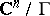, usually known as uniformization, over such fields, was constructed by D. Mumford and M. Raynaud. Unlike the complex case, not all Abelian varieties, but only those having a reduction to a multiplicative group modulo, are uniformizable . The theory of Abelian varieties over global (number and function) fields plays an important role in Diophantine geometry. Its principal result is the Mordell–Weil theorem: The group of rational points of an Abelian variety, defined over a finite extension of the field of rational numbers, is finitely generated.